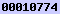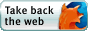# fundest: A C/C++ Library for Robust, Non-linear Fundamental Matrix Estimation

Manolis Lourakis
Institute of Computer Science,
Foundation for Research and Technology - Hellas,
Heraklion, Crete, Greece

Last updated Feb. 14, 2016

*NEW*: version 1.2 is out; see the change log.

### Introduction

This page concerns fundest, a C/C++ library for fundamental matrix estimation that is distributed under the GNU General Public License (GPL). The fundamental matrix is a singular 3x3 matrix which relates corresponding points in two views according to the epipolar constraint. A fundamental matrix has rank two and is defined up to an unknown scale, hence has seven degrees of freedom. fundest implements a technique for non-linear, robust fundamental matrix estimation from matched image point features. This technique computes an estimate that minimizes an appropriate cost function defined on matching points (currently either algebraic error with rank-2 enforcement, symmetric distances from epipolar lines or Sampson error) and includes robust regression techniques for coping with outliers. Briefly, the algorithm implemented by fundest is the following:

1. Normalization of point coordinates to improve conditioning as described in R.I. Hartley “In Defense of the Eight-Point Algorithm”, IEEE Trans. on PAMI, Vol. 19, No. 6, pp. 580-593, Jun. 1997.

2. Least Median of Squares (LMedS) linear fitting with the eight-point algorithm to detect outliers, see P. Rousseeuw, “Least Median of Squares Regression”, Journal of the American Statistics Association, Vol. 79, No. 388, pp. 871-880, Dec. 1984. To ensure adequate spatial distribution of point quadruples over the image, LMedS random sampling employs the bucketing technique proposed in Z. Zhang, R. Deriche, O. Faugeras, Q.T. Luong, “A Robust Technique for Matching Two Uncalibrated Images Through the Recovery of the Unknown Epipolar Geometry”, Artificial Intelligence, Vol. 78, Is. 1-2, pp. 87-119, Oct. 1995. Also published as INRIA RR-2273, May 1994.
The rank-2 contraint is enforced a posteriori on the estimated matrix by setting its smallest singular value to zero.

3. Non-linear refinement of the fundamental matrix linear estimate by minimizing one of the cost functions listed below (at the user's choice and in increasing order of computational overhead).
The algebraic error is minimized by varying the left epipole and is performed in way that intrinsically respects the rank-2 constraint. In the Sampson error and symmetric distance cases, the rank-2 constraint is enforced by parametrizing the fundamental matrix so that one of its rows is expressed as a linear combination of the other two.

In all cases, the minimization is performed using the Levenberg-Marquardt algorithm, see M.I.A. Lourakis, “levmar: Levenberg-Marquardt Nonlinear Least Squares Algorithms in C/C++”, [web page] http://www.ics.forth.gr/~lourakis/levmar/.

To cope with degenerate data, model selection using the so-called Geometric Robust Information Criterion (GRIC) is included to facilitate the assesment of the likelihood of a fundamental matrix fit vs. that of a homography. The latter is estimated with homest and requires the symbol HAVE_HOMEST to be defined during compilation.

More details on fundamental matrix estimation can be found in chapters 9 & 11 of R. Hartley and A. Zisserman's textbook titled “Multiple View Geometry in Computer Vision”, Cambridge University Press, 2003. Note that fundest does not include any means for detecting and matching point features between images. Such functionality can be supplied by other software such as D. Lowe's SIFT detector & descriptor, the VLFeat and OpenSURF libraries or, in some cases, the KLT tracker.

To compile, fundest requires the levmar Levenberg-Marquardt non-linear least squares package, which in turn relies on BLAS/LAPACK. Precompiled LAPACK/BLAS binaries for Windows are available here and here.

The source code is distributed in a gzip'ed tar file. It has been tested under linux with gcc/lcc and under windows with MSVC but should compile with any ANSI compliant C compiler. Remember to include fundest.h from your C/C++ code.

Current version: fundest-1.2

For historical reasons, older versions of fundest are also available:hits since Sat Jun 30 23:40:15 EEST 2012